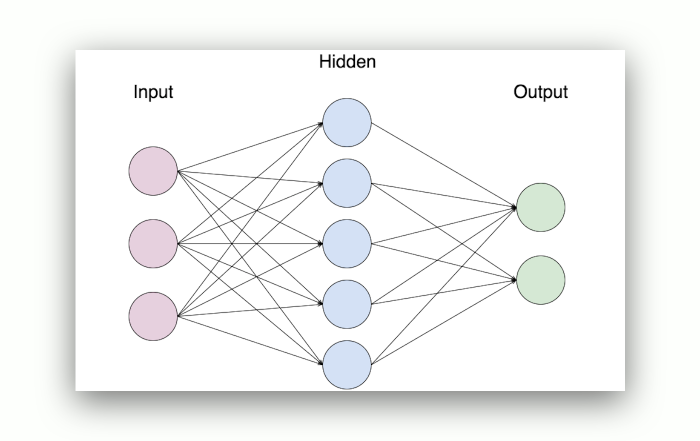Neural networks are inspired by the brain. The model has many neurons (often called nodes). We don’t need to go into the details of biology to understand neural networks.

Like a brain, neural networks can “learn”. Instead of learning, the term “training” is used. If training is completed, the system can make predictions (classifications).

Related Course:
Deep Learning for Computer Vision with Tensor Flow and Keras## Introduction

The neural network has: an input layer, hidden layers and an output layer. Each layer has a number of nodes.

The nodes are connected and there is a set of weights and biases between each layer (W and b).

There’s also an activation function for each hidden layer, σ. You can use the sigmoid activation function.When couting the layers of a network, the input layer is often not counted. If we say 2-layer neural network, it means th
ere are 3 layers.

To explain better, we’ll add some sample code in this tutorial.

In code:

## Layers

The layers are connected. The first layer is made with the input layer and weights. In this case the output layer is made with layer1 and weights2.

For a 2 layer neural network, you can have this:

## Training

Remember we said neural networks have a training process?

The training process has multiple iterations. Each iteration

• calculate the predicted output y (feedforward)
• updates the weights and biases (backpropagation)

During the feedforward propagation process (see code above), it uses the weights to predict the output.
But what is a good output?

To find out, you need a loss function (frequently called cost function). There are many loss functions.

The loss function will be used to update the weights and biases. It’s part of the backpropagation process.